# Tutorials, applications

These tutorials present real-life applications of the `pykeops.numpy` and `pykeops.torch` modules, from Gaussian processes to Optimal Transport theory and K-means clustering.

Note

If you run a KeOps script for the first time, the internal engine may take a few minutes to compile all the relevant formulas. Do not worry: this work is done once and for all as KeOps stores the resulting shared object files (‘.so’) in a cache directory.

## KeOps 101: Working with LazyTensors

Thanks to the `pykeops.numpy.LazyTensor` and `pykeops.torch.LazyTensor` decorator, KeOps can now speed-up your NumPy or PyTorch programs without any overhead.A wrapper for NumPy and PyTorch arrays

A wrapper for NumPy and PyTorch arraysFancy reductions, solving linear systems

Fancy reductions, solving linear systemsAdvanced usage: Vi, Vj, Pm helpers and symbolic variables

Advanced usage: Vi, Vj, Pm helpers and symbolic variablesTensorDot

TensorDot

## Using KeOps as a backend

KeOps can provide efficient GPU routines for high-level libraries such as scipy or GPytorch: if you’d like to see tutorials with other frameworks, please let us know.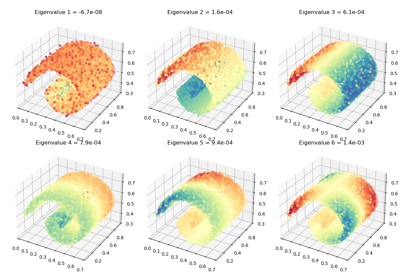## Gaussian Mixture Models

KeOps can be used to fit Mixture Models with custom priors on large datasets: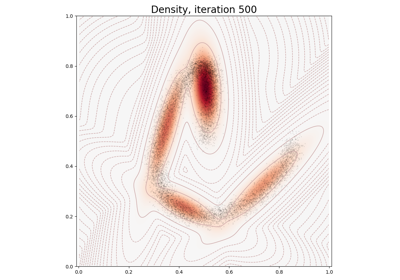Fitting a Gaussian Mixture Model

Fitting a Gaussian Mixture Model

## Interpolation - Splines

Thanks to a simple conjugate gradient solver, the `numpy.KernelSolve` and `torch.KernelSolve` operators can be used to solve large-scale interpolation problems with a linear memory footprint.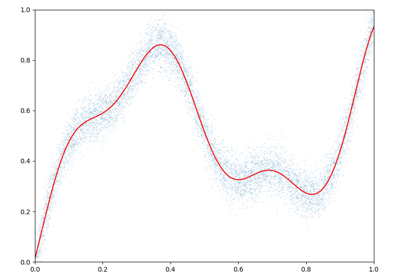Kernel interpolation - NumPy API

Kernel interpolation - NumPy API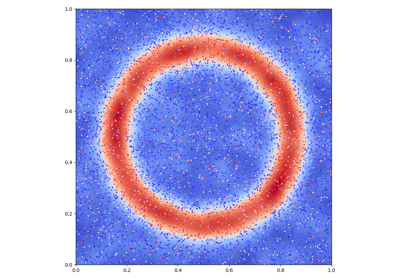Kernel interpolation - PyTorch API

Kernel interpolation - PyTorch API

## K-means

A fast implementation of the K-means algorithm on the GPU.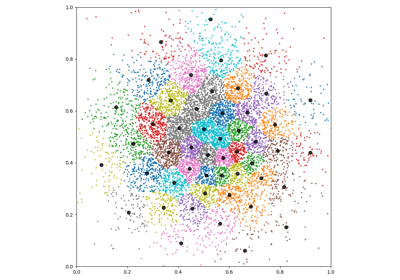K-means clustering - NumPy API

K-means clustering - NumPy API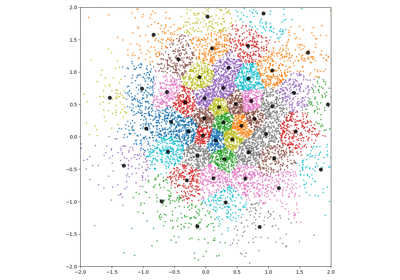K-means clustering - PyTorch API

K-means clustering - PyTorch API

## K-Nearest Neighbors

K-NN classification using the ArgKMin reduction.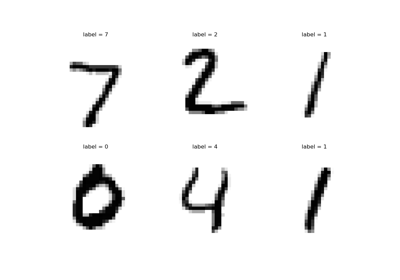K-NN on the MNIST dataset - PyTorch API

K-NN on the MNIST dataset - PyTorch API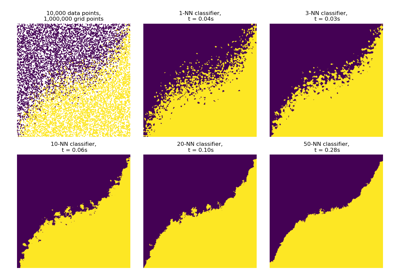K-NN classification - NumPy API

K-NN classification - NumPy API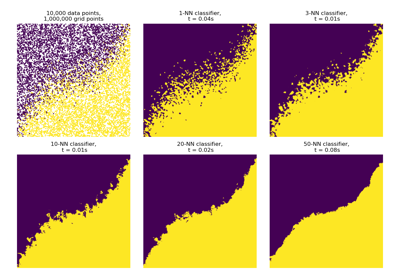K-NN classification - PyTorch API

K-NN classification - PyTorch API

## Kernel MMDs, Optimal Transport

Thanks to its support of the Sum and LogSumExp reductions, KeOps is perfectly suited to the large-scale computation of Kernel norms and Sinkhorn divergences. Going further, the block-sparse routines allow us to implement genuine coarse-to-fine strategies that scale (almost) linearly with the number of samples, as advocated in (Schmitzer, 2016).

Relying on the KeOps routines `generic_sum()` and `generic_logsumexp()`, the GeomLoss library provides Geometric Loss functions as simple PyTorch layers, with a fully-fledged gallery of examples. Implemented on the GPU for the very first time, these routines outperform the standard Sinkhorn algorithm by a factor 50-100 and redefine the state-of-the-art for discrete Optimal Transport: on modern hardware, Wasserstein distances between clouds of 100,000 points can now be computed in less than a second.

## Surface registration

Diffeomorphic registration of meshes with the LDDMM framework.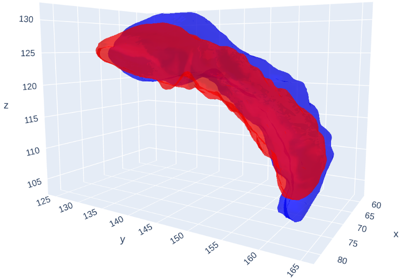Surface registration

Surface registration

Gallery generated by Sphinx-Gallery